# Hack 66. Play a Tune on Pascal s Triangle

### Hack 66. Play a Tune on Pascal's Triangle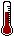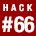Need to know the odds quickly? Pascal's Triangle is a simple layout of numbers that allows for quick and easy calculations of probability. It's worked for 300 years, so I bet it will work for you.

The thing that statisticians do most often is calculate probabilities, which can describe expected outcomes for a variety of situations. A simple example is flipping a coin. Imagine that you have been asked to wager on the outcome of a coin flip. With two possible outcomes, heads or tails, the chances of getting either outcome on a single coin flip is 1 out of 2, or 1/2.

The math is easy if you know the number of different ways to get the winning outcome and the number of possible outcomes. In the coin flip example, there's only one way to get a winning outcome, and there are only two possible outcomes. The math is just a bit harder if we have more than one coin flip and wonder about the number of all possible outcomes and how many of those combinations would match our winning criteria. For example, if I want two heads in a row on two coin flips, I could list all possible outcomes, identifying the number of those outcomes that make me a winner, and then see what proportion of all outcomes are winners for me. That proportion would be my chances of winning.

The number of possible outcomes that count as winners is often more complex than our simple coin flip examples, though, because there might be many trials (or dice rolls, or purchase of lottery tickets, or whatever) and many different combinations. For example, you might want to figure the number of possible combinations of different elements from any set of objects you're drawing out of a hat or choosing through some other random selection process.

Imagine you are one of six relatives driving to the airport and you must all drive there in one big van. None of you like each other much, so you need some fair way to decide who will sit where. You will randomly pick two names to drive together in the front seat.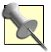A private note to my Uncle Frank: yes, this example is based on the "unpleasantness" last Thanksgiving. All is forgiven, at least on my side of the family, but we agree it would be best if you brought your own car next year.

Now, you need to know the chances that you will be in the front seat and who you might be with. The problem is calculating how many different combinations of relatives could be in that front seat. For both simple wagers, such as coin flips, and life-and-death situations, such as long car trips, you can use a layout of numbers called Pascal's Triangle to do the math for you.

#### Presenting Pascal's Triangle

Pascal's Triangle is shown in Figure 6-6. This layout of numbers has some interesting properties. Here it is shown made up of 10 rows, with 10 numbers in its lowest row, but it can be made infinitely large with an infinite number of rows. The outer edges going down are all 1s. The next diagonals start with 1 but increase by 1 as they go down.

##### Figure 6-6. Pascal's Triangle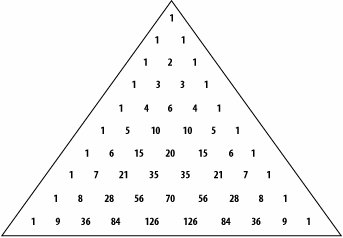Similar interesting progressions are found throughout the Triangle. Notice that each number is the sum of the two numbers above it: 84 is 56 + 28, 7 is 6 + 1, and so on. These cool patterns aren't the reason that the Triangle is of interest to us, however. Instead, we're going to use it to calculate the probabilities for a variety of outcomes.

#### Calculating Probability Using the Triangle

Pascal's Triangle, named for Blaise Pascal (a very smart, very early contributor to probability theory who lived in the 1600s), has already made use of all the calculations we need to answer a variety of questions.Though this pattern of numbers is known as Pascal's Triangle, Pascal did not, and never claimed to have, originated it. Similar patterns of numbers were presented by Pascal's teacher, H\x8e rigone, as well as other colleagues writing at about the same time.

A general formula exists to determine the number of possible outcomes of a certain type. This general formula works any time there are exactly two possible outcomes; thus, the term binomial coefficient is used to describe the outcome of the formula (bi-nomial means having two names or, in a statistical sense, two outcomes). To determine the number of possible binomial combinations of outcomes across a given number of trials, this formula is used:

A range of possible values that could be entered into this formula are the coordinates on Pascal's map. The n in the equation, which represents the number of trials or events, indicates which row to go to. The k in the equation tells us which entry on that row to go to. The 1s along the left of the map are like a border: they count as zero. So, to use the triangle, we start counting at 0.The exclamation point after some numbers in this formula means factorial, which, in turn, means that you are supposed to count down from that number to 1, multiplying the numbers together as you go. For example, 5 factorial means 5x4x3x2x1, or 120. By the way, by rule, 0! counts as 1.

##### Assessing the probability of flipped coin outcomes

For our second, slightly more complex coin flip question, the chances of getting exactly two heads when flipping a coin two times, use the triangle like this:

1. The row to go to is determined by the number of coin flips we will make: 2. The entry we will count over to in that row is determined by the number of heads we want to see: 2. With our coin flip example, 2 heads on 2 trials, count down two rows to this row:

2. 1 2 1

3. Then, count over two entries to the 1. Our answer is 1, and so there is one chance that we will get two heads.

4. But 1 chance out of how many chances? Add up all the numbers in the row you are in to get that answer! 1 + 2 + 1 = 4, so our chances are 1 out of 4, or 25 percent.

The Triangle answers more complicated question, as well. Suppose you want exactly 3 heads out of six coin flips:

1. Count down six rows (remember to count the top of the triangle as 0). You get to this row:

2. 1 6 15 20 15 6 1

3. Count over three numbers and you hit 20. There are 20 different ways that exactly three heads could come up in six coin flips.

4. 20 out of how many possibilities, you ask? Summing all the values in that row gives us 64. 20 out of 64 times you will get exactly three heads (or three tails). That's about 31 percent of the time.

##### Assessing the probability of a bad car trip

Another way to use the triangle is to see how many combinations are possible from a certain number of elements drawn out in a certain way. Our car trip example is interested in how many possible combinations of two people can be drawn from a group of six.

From a set of six elements, you will draw out two of them and match those up. For this question, and in the binomial formula that defines the triangle, think of the six relatives as n and the two names to be drawn as the k:

1. Count down six rows and across two entries, and you hit the number 15. There are 15 possible combinations of two people drawn from six people.

2. In this case, you are interested only in your chances of being in the front seat with one specific person. That's 1 combination of front seat passengers out of 15 possible combinations. So, you will be matched up with your annoying Uncle Frank, or Aunt Tillie, or whomever, in the front seat just 1 out of 15 times.

#### Why It Works

The numbers in the triangle match the values that you would derive if you actually did the math using the binomial equation, but you'll notice that the triangle answers several other questions along the way. The patterns of the numbers, their progression, are consistent with other formulas used in determining probability.

For instance, the total possible number of coin flip combinations for six coin flips is answered on the triangle by totaling the values in the sixth row: 64. You would have mathematically derived that value by applying the general formula for number of possible outcomes for a coin: 2number of flips = 26 = 64.

As for the chances that you would both be chosen as one of two people out of six and that the other person would be a specific one of the other people (our trip to the airport example), the triangle said 1 out of 15. But you also could have figured it this way:

1. Chance of being in a group of two people out of six = 2/6 = .33

2. Chance of a specific "other" person being chosen = 1 person out of 5 "others" = 1/5 = .20

3. Chance of both outcomes occurring = .33x.20 = .066, and .066 = 1 out of 15

So, when you have a complicated-looking problem that involves combinations and permutations and so many possibilities that it makes your head spin, let the soothing music of Pascal's Triangle bring peace to your troubled mind.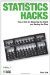Statistics Hacks: Tips & Tools for Measuring the World and Beating the Odds
ISBN: 0596101643
EAN: 2147483647
Year: 2004
Pages: 114
Authors: Bruce Frey

Similar book on Amazon